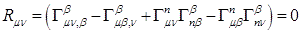## Pages

### 16. Schwarzchild Solution (cntd..)

SOLVING THE VACUUM FIELD EQUATION
Let us assume a sphere of gravitating mass $M$ assumed to be at rest. We place this mass at the origin of our coordinate system. For convenience, we will use a spherical coordinates$\rho,\theta,\phi$; we then have the following transformation rule,
$x=\rho\sin\theta\cos\phi$,  $x=\rho\sin\theta\sin\phi$,  $z=\rho\cos\theta$

In solving the vacuum field equation Karl Schwarzschild made the following assumption,
1.    A spherically symmetric spacetime in which all metric components are unchanged under any rotation-reversal $\theta\rightarrow -\theta$ or $\phi\rightarrow -\phi$.
2.    A static spacetime in which all metric components are independent of the time coordinate $t$ (so that $(\partial g_{\mu\nu}/\partial t )=0$ and the geometry of spacetime is unchanged under a time-reversal $t \rightarrow -t$.

We know that the invariant interval of Minkowskian spacetime in special relativity written in spherical coordinates is as follows,
$ds^{2}=-dt^{2}+d\rho^{2}+\rho^{2}d\theta^{2}+\rho^{2}\sin^{2}\theta d\phi^{2}$                                  $(1)$

But in general, the spacetime is spherically symmetric if every point in the spacetime lies on a $2-D$ surface which is a $2$-sphere. Using spacetime coordinates $(t,\rho,\theta,\phi)$, then the interval is

$dl^{2}=f(\rho ,t)[d\theta ^{2}+\sin ^{2}\theta d\phi ^{2}]$

Where, $t$ and $\rho$ are constant in case if $2$-sphere geometry and $\sqrt{f(\rho ,t)}$ is the radius of the curvature of $2$-sphere.

In case of the flat spacetime, the radius of curvature is found to be the radial spherical coordinate $\rho$, but in curved spacetime the relationship between the angular coordinates of the $2$-sphere and the remaining coordinates is not always simple for each point in spacetime. Nevertheless, we can always define a new radial coordinate,$r$ which satisfies $r^{2}= {f(\rho ,t)}$.

Now we know that the differential length in spacetime is related to the metric as follows,

$ds^{2}=g_{\mu\nu}dx^{\mu}dx^{\nu}$

And from the assumption of the static and spherically symmetric spacetime our metric is subjected to constraint in the angular and the temporal coordinates. Thus, it is not difficult to show that our metric $g_{\mu\nu}$ has only the diagonal terms.
If we label our system of coordinate as, $x^{0}=t$, $x^{1}=r$, $x^{2}=\theta$, $x^{3}=\phi$ then,

We may now write the general form of the invariant interval for static spherically symmetric spacetime as,
$ds^{2}= g_{tt}dt^{2}+ g_{rr}dr^{2}+ g_{\theta\theta}d\theta^{2}+ g_{\phi\phi}d\phi^{2}$

Now from equation $(1)$ we can assume that the general form that the above metric takes in the flat spacetime to be as follows,
$ds^{2}=-A(r)dt^{2}+ B(r)dr^{2}+r^{2}d\theta^{2}+r^{2}\sin^{2}\theta d\phi^{2}$

Here, we have redefined our radial coordinate as $r^{2}=f(\rho)$.

Now for mathematical convenience we also assume that the coefficient to be some function of the exponential. We have then to rewrite the coefficient as, $A(r)=e^{2m}$ and $B(r)=e^{2n}$ .
Our equation then becomes,
$ds^{2}=- e^{2m}dt^{2}+ e^{2n}dr^{2}+r^{2}d\theta^{2}+r^{2}\sin^{2}\theta d\phi^{2}$                        $(2)$

We must keep in mind that the exponential terms are still the function of the radial coordinates.

Since our system of coordinate has been written as, $x^{0}=t$, $x^{1}=r$, $x^{2}=\theta$, $x^{3}=\phi$, then from $(2)$ the metric tensor is as follows,

We now have to solve for the values of $-e^{2m}$ and $e^{2n}$. For that we must use the Ricci Tensor equation from the vacuum field equation,
$R_{\mu\nu}=0$We also recall that,
Now since,$g_{\mu\nu}$ for $\mu\neq\nu$ and as ,$g^{\mu\nu}=1/g_{\mu\nu}$ so we can simplify the above Christoffel symbol as,

Now we have to evaluate the Christoffel symbols, however we will not be evaluating the whole process but instead write the final field equation after we have evaluated the Christoffel symbol.
So, after we evaluate Christoffel symbol we can rewrite the field equation as follows,

Remember that we have used the result from the mathematical discussion in the previous section.

Now we must remind ourselves that the method for evaluating the Ricci tensor requires a little hard work and a good amount of concentration. In this discussion here, we only state the final result of the evaluation. So, evaluating the Ricci tensor we get the following;
for  $\mu=\nu$,
for  $\mu\neq\nu$,
$R_{\mu\nu}=0$
Now, from the vacuum field equation, the individual term in the Ricci tensor must be zero, we then have the following set of equation,

So, these are the final equation that we have to solve.
From adding $(3)$ & $(4)$ and applying the boundary condition that as $r\rightarrow \infty e^{2m}\rightarrow 1$ and $e^{2n}\rightarrow 1$ we get,

$m+n=0$                                                               $(7)$

Substituting equation $(7)$ in $(5)$ we get the following,

Thus, this implies after integrating with respect to $r$,
for some constant $\alpha$.
To find $\alpha$ we will use the geodesics equation on a test particle which is initially at rest. We have the geodesic equation as,

Now since, we release particle which is at rest we can rewrite $(2)$ as follows,

$ds^{2}=- e^{2m}dt^{2}+0+0+0$

We also know that for time like intervals, we can relate proper time $\tau$ between two events as,
This implies from above two equations, $(\partial t/\partial \tau)=(c/e^{m})$
Recall that we are attempting to solve for $\alpha$ and that we want our metric to reduce to Newtonian gravitation at the weak field limit. Let us find the geodesic equation for $x^{\lambda}=x^{1}=r$ at the instant we release our test particle. Remember that we are releasing this particle from rest and so $(d x^{\lambda}/d \tau)$for ${\lambda}\neq 0$ and so we are left with only following in our summation

Substituting $(\partial t/\partial \tau)=(c/e^{m})$, $\Gamma _{00}^{1}$ and comparing it with the Newtonian gravitational acceleration, we get the following,
Thus we have,
$\alpha=-2GM$

Finally we now substitute $\alpha$ in equation $(8)$ and then substituting equation $(8)$ in equation $(2)$ we have the required solution,

Hence, we have found a metric in a vicinity of mass where spacetime is spherically symmetric and static. This metric is also called the Schwarzschild metric.

This solution was first found by Karl Schwarzschild and it is called the Schwarzschild solution.